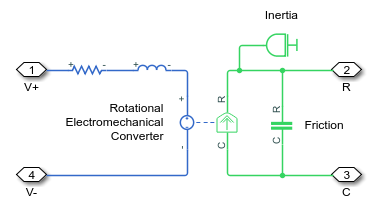# subsystem2ssc

Convert subsystem containing Simscape blocks into equivalent Simscape file or files

## Syntax

``subsystem2ssc(subsystem)``
``subsystem2ssc(subsystem,targetFolder)``

## Description

example

````subsystem2ssc(subsystem)` converts a subsystem consisting entirely of Simscape™ blocks into a single Simscape component file, located in the current working folder. The function generates a composite component file based on the subsystem configuration. If the subsystem being converted contains nested subsystems, then the function generates several Simscape files, one for each subsystem.You can mark member block and subsystem parameters for promotion to the top level, and the function automatically generates the corresponding code, similar to composite components. For more information, see Parameter Promotion.The subsystem being converted cannot contain blocks from the Simscape Utilities library (such as Solver Configuration, PS-Simulink Converter, Simulink-PS Converter, Simscape Bus, and so on) because they are not authored in Simscape language and therefore have no equivalent textual representation. The exception is the Connection Port block, because it can be represented by the `connect` statements in Simscape language. If the subsystem contains a Simscape Component block, then during the conversion this block is replaced by its source component.```

example

````subsystem2ssc(subsystem,targetFolder)` converts a subsystem containing Simscape blocks into equivalent Simscape component file, or files, located in `targetFolder`.```

## Examples

collapse all

Open the Permanent Magnet DC Motor example model. At the MATLAB® command prompt, enter:

`openExample('simscape/PermanentMagnetDCMotorExample')`This example model contains a subsystem named DC Motor. Convert this subsystem into a Simscape component file and place this file in your current working folder.

```subsystem2ssc('PermanentMagnetDCMotor/DC Motor') ```

The function creates a file named `DC_Motor.ssc` in the current folder. Double-click the `DC_Motor.ssc` file to open it in the editor.

```component (Propagation = blocks) DC_Motor nodes C = foundation.mechanical.rotational.rotational; R = foundation.mechanical.rotational.rotational; V1 = foundation.electrical.electrical; V0 = foundation.electrical.electrical; end components(ExternalAccess = observe) Rotor_Resistance = foundation.electrical.elements.resistor(R = {3.9, 'Ohm'}); Rotor_Inductance = foundation.electrical.elements.inductor(g = {1e-09, '1/Ohm'}, l = {1.2e-05, 'H'}, r = {0, 'Ohm'}, i_L = {value = {0, 'A'}, priority = priority.high}); Rotational_Electromechanical_Converter = foundation.electrical.elements.rotational_converter(K = {.0006875493541569879, 'V*s/rad'}); Inertia = foundation.mechanical.rotational.inertia(inertia = {.01, 'cm^2*g'}, num_ports = int32(1)); Friction = foundation.mechanical.rotational.friction(Col_trq = {2e-05, 'N*m'}, brkwy_trq = {2e-05, 'N*m'}, brkwy_vel = {.03347, 'rad/s'}, visc_coef = {0, 'N*m*s/rad'}); end connections connect(V0,Rotor_Resistance.p); connect(Rotational_Electromechanical_Converter.p,Rotor_Inductance.n); connect(V1,Rotational_Electromechanical_Converter.n); connect(Rotor_Inductance.p,Rotor_Resistance.n); connect(R,Friction.R); connect(R,Inertia.I); connect(R,Rotational_Electromechanical_Converter.R); connect(C,Friction.C); connect(C,Rotational_Electromechanical_Converter.C); end end```

Double-click the DC Motor subsystem in the example model and compare it to the generated file.The generated composite component contains the same components, parameters, and connections as the original subsystem.

The `(Propagation = blocks)` attribute is automatically added to converted graphical subsystems. For this particular subsystem, it does not make any difference. But, for example, if a subsystem contains blocks from two separate fluid networks, setting `(Propagation = blocks)` prevents compilation errors if the two networks have different domain parameters. For more information, see Model Attributes.

Open the Permanent Magnet DC Motor example model. At the MATLAB command prompt, enter:

`openExample('simscape/PermanentMagnetDCMotorExample')`This example model contains a subsystem named DC Motor. Convert this subsystem into a Simscape component file and place the generated file in a designated folder.

```subsystem2ssc('PermanentMagnetDCMotor/DC Motor','./MotorsLibrary') ```

The function creates a file named `DC_Motor.ssc` and places it into the folder named `MotorsLibrary`.

If the specified target folder does not exist, the function creates it.

## Input Arguments

collapse all

Subsystem name or identifier, specified as a block handle or a full name, including the path to the subsystem from the root of the model.

Example: 'PermanentMagnetDCMotor/DC Motor'

Data Types: `double` | `char` | `string`

Location of generated component files, specified as a character vector or string scalar. The function places the generated component files in this folder. If the folder does not exist, the function creates it.

Example: './files'

Data Types: `char` | `string`

## Version History

Introduced in R2018b